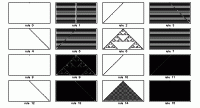# Long-Range Cellular Automata

(This post was originally published on the NKS Forum.)

At the NKS 2004 conference I did my now-traditional “live computer experiment”. This time the topic I picked came from a question someone asked at the minicourse before the conference: does increasing the “range” of a cellular automaton have a big effect on its behavior?

I decided to investigate a simple version of this question.

In an ordinary r=1 cellular automaton, the new color of a particular cell depends on the previous colors of cells with offsets -1, 0, 1. The question I asked was then: what happens if the offsets are larger?

In the simplest non-trivial cellular automata, the color of a cell depends on the previous colors of two cells. In the ordinary short-range case, the cells have offsets -1, 1. But now we can ask what happens if instead they have offsets -1, m.

It’s easy to look at this with the built-in CellularAutomaton function in Mathematica. You just use CellularAutomaton[{n, k, {{-1}, {m}}}, init, t], where n is the rule number, and k is the number of colors. (The offsets get put in sublists to distinguish them from multidimensional range specifications.)

With k=2 colors, there are 16 such rules. The example below shows the usual short-range case of offsets -1, 1. The most complex pattern here is a nested one, corresponding to elementary rule 90 (which here is rule number 6).The images below show the cases of offset -1, 2 (on the left) and -1, 3 (on the right). The pictures are distorted, but fundamentally exactly the same as the -1, 1 case.

It’s not surprising that this happens. In the usual -1, 1 case, there are already two independent sublattices: even cells on even steps, and odd cells on even steps. In the -1, m case, there are m+1 sublattices, but each one has exactly the same dependence structure as the sublattices in the -1, 1 case, just progressively shifted. (This is particularly obvious in the case of the checkerboard pattern of rule 14.)

So what about the three-cell-dependence case? Offsets -1, 0, 1 (with k=2 colors) give the usual 256 elementary cellular automata, shown in the top example. But now the second and third examples give the -1, 0, 2 and -1, 0, 3 cases respectively.

What happens is rather interesting. Many -1, 0, m rules give qualitatively similar patterns when m=2 or m=3 as when m=1. There are lots of detailed differences, though. And a number of rules that are somehow “incipiently complex” when m=1 (like 22, 41 and 54) become more obviously complex for m=2. An example is rule 22, which for m=1 yields a nested pattern when there is a single black initial cell, but a more complicated pattern with certain larger initial conditions (see the NKS book, page 263).

Rules that do not depend on all three neighbors also show simple shifting, just like in the -1, m case. Additive rules, like 90 and 150, always give nested patterns for any offsets. Those for rule 90 are just shifted. But for rule 150, they have progressively different forms (see examples below). One can nevertheless still compute their fractal dimensions using the program from the NKS book, page 955; the results for m=1 through 5 are {1.69, 1.727, 1.736, 1.745}.

It’s interesting to see what happens with, say, rule 30, as one increases the offset (see examples below). There are some definite surprises. In particular, for some m (notably, multiples of 4) the pattern for rule 30 seems to get much coarser. At first, it might look like its center column repeats. But it doesn’t seem to.

At NKS 2004, I spent an hour looking at all of this—with some help from the audience. It was hard to stop… The raw notebook I produced from the session is 48 megabytes. Here I’ve attached just the small notebook (Inputs.nb) of inputs.

There are lots and lots of obvious things to investigate with long-range cellular automata. What makes particular offsets lead to coarser patterns? What determines their coarseness? What overall growth rates occur for different offsets? What happens if one uses different sets of offsets? (-m, 0, m just give stretched versions of -1, 0, 1 patterns, but what about others?) Even more, what happens if one uses offsets in time, as well as in space (compare page 437)? There are lots of interesting specific questions about particular rules too. Like what’s going on with the right-hand edge of rule 121 for m>1?